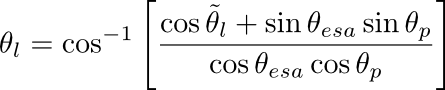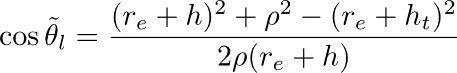## Calibration

The complex amplitude for each channel is divided by the square root of the area correction factor, Ao, computed with the following logic (each quantity below may be found in the annotation file):

ρ     --  Range to pixel (m) (computed from "Image Starting Range" and "Range Spacing per Bin")
h     --  global average alitude (m)
ht    --  global average terrain height (m)
Δρ   -- range spacing per bin(m)
Δaz  -- azimuth spacing (m)
re     -- the local radius of curvature of earth "Ellipsoid Semi-major Axis" (m)
θesa  -- electronic steering angle "Global Average ESA" (radians)
θp    -- global average pitch of aircraft (radians).where:then:The units of the data in all cases is linear rather than db units.